Olympiad Test: Embedded Figure - 1

# Olympiad Test: Embedded Figure - 1

Test Description

## 10 Questions MCQ Test Mathematical Olympiad Class 8 | Olympiad Test: Embedded Figure - 1

Olympiad Test: Embedded Figure - 1 for Class 8 2022 is part of Mathematical Olympiad Class 8 preparation. The Olympiad Test: Embedded Figure - 1 questions and answers have been prepared according to the Class 8 exam syllabus.The Olympiad Test: Embedded Figure - 1 MCQs are made for Class 8 2022 Exam. Find important definitions, questions, notes, meanings, examples, exercises, MCQs and online tests for Olympiad Test: Embedded Figure - 1 below.
Solutions of Olympiad Test: Embedded Figure - 1 questions in English are available as part of our Mathematical Olympiad Class 8 for Class 8 & Olympiad Test: Embedded Figure - 1 solutions in Hindi for Mathematical Olympiad Class 8 course. Download more important topics, notes, lectures and mock test series for Class 8 Exam by signing up for free. Attempt Olympiad Test: Embedded Figure - 1 | 10 questions in 20 minutes | Mock test for Class 8 preparation | Free important questions MCQ to study Mathematical Olympiad Class 8 for Class 8 Exam | Download free PDF with solutions
 1 Crore+ students have signed up on EduRev. Have you?
Olympiad Test: Embedded Figure - 1 - Question 1

### Direction: In each of the following questions, fig. (X) is embedded in any one of the four alterative figures (a), (b), (c) or (d). Find the alternative which contains fig. (X) as its part.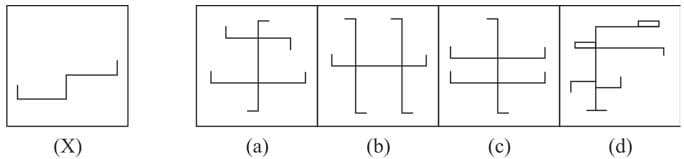Olympiad Test: Embedded Figure - 1 - Question 2

### Direction: In each of the following questions, fig. (X) is embedded in any one of the four alterative figures (a), (b), (c) or (d). Find the alternative which contains fig. (X) as its part.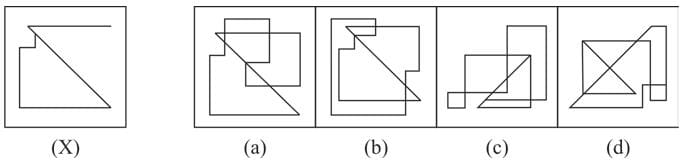Olympiad Test: Embedded Figure - 1 - Question 3

### Direction: In each of the following questions, fig. (X) is embedded in any one of the four alterative figures (a), (b), (c) or (d). Find the alternative which contains fig. (X) as its part.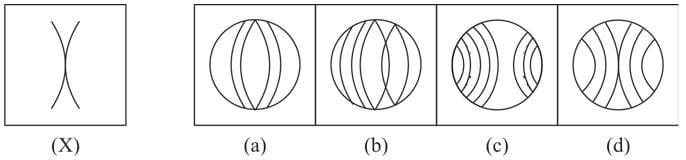Olympiad Test: Embedded Figure - 1 - Question 4

Direction: In each of the following questions, fig. (X) is embedded in any one of the four alterative figures (a), (b), (c) or (d). Find the alternative which contains fig. (X) as its part.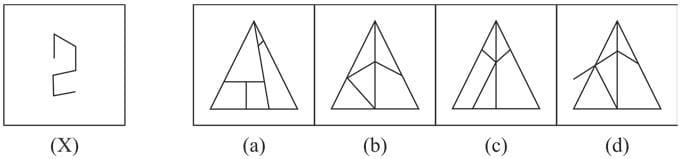Olympiad Test: Embedded Figure - 1 - Question 5

Direction: In each of the following questions, fig. (X) is embedded in any one of the four alterative figures (a), (b), (c) or (d). Find the alternative which contains fig. (X) as its part.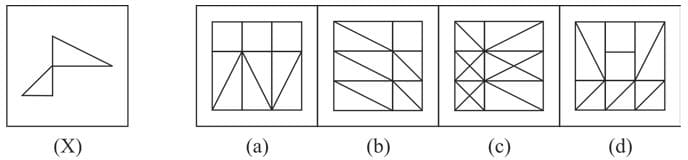Detailed Solution for Olympiad Test: Embedded Figure - 1 - Question 5

Solution :

The correct option is Option A.

Clearly, figure X is embedded in option A.

Olympiad Test: Embedded Figure - 1 - Question 6

Direction: In each of the following questions, fig. (X) is embedded in any one of the four alterative figures (a), (b), (c) or (d). Find the alternative which contains fig. (X) as its part.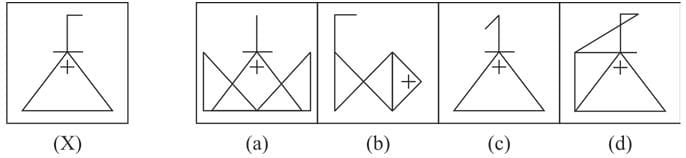Olympiad Test: Embedded Figure - 1 - Question 7

Direction: In each of the following questions, fig. (X) is embedded in any one of the four alterative figures (a), (b), (c) or (d). Find the alternative which contains fig. (X) as its part.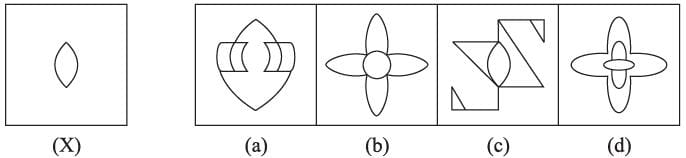Olympiad Test: Embedded Figure - 1 - Question 8

Direction: In each of the following questions, fig. (X) is embedded in any one of the four alterative figures (a), (b), (c) or (d). Find the alternative which contains fig. (X) as its part.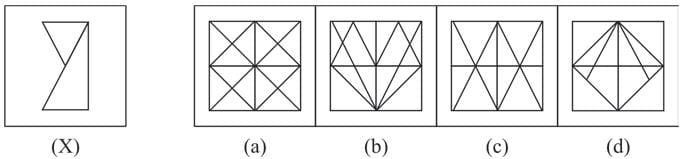Olympiad Test: Embedded Figure - 1 - Question 9

Direction: In each of the following questions, fig. (X) is embedded in any one of the four alterative figures (a), (b), (c) or (d). Find the alternative which contains fig. (X) as its part.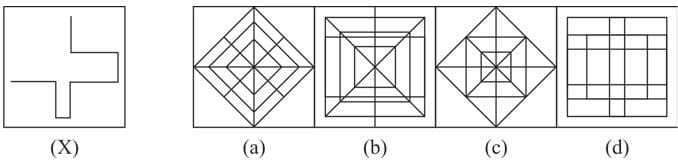Olympiad Test: Embedded Figure - 1 - Question 10

Direction: In each of the following questions, fig. (X) is embedded in any one of the four alterative figures (a), (b), (c) or (d). Find the alternative which contains fig. (X) as its part.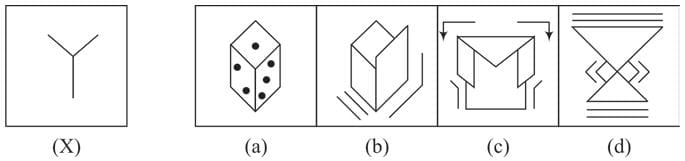## Mathematical Olympiad Class 8

53 tests
 Use Code STAYHOME200 and get INR 200 additional OFF Use Coupon Code
Information about Olympiad Test: Embedded Figure - 1 Page
In this test you can find the Exam questions for Olympiad Test: Embedded Figure - 1 solved & explained in the simplest way possible. Besides giving Questions and answers for Olympiad Test: Embedded Figure - 1, EduRev gives you an ample number of Online tests for practice

53 tests